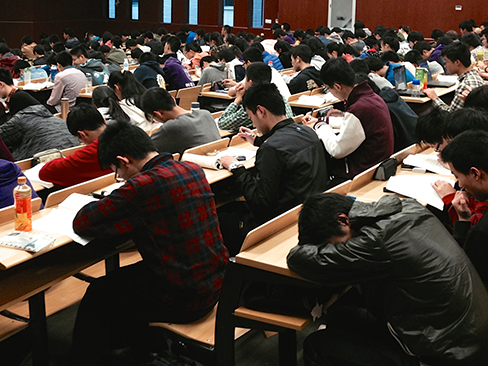## Models and Applications

### Learning Outcomes

• Write a linear equation to express the relationship between unknown quantities.
• Write a linear equation that models two different cell phone packages.
• Use a linear model to answer questions.
• Set up a linear equation involving distance, rate, and time.
• Find the dimensions of a rectangle given the area.
• Find the dimensions of a box given information about its side lengths.

Josh is hoping to get an A in his college algebra class. He has scores of 75, 82, 95, 91, and 94 on his first five tests. Only the final exam remains, and the maximum of points that can be earned is 100. Is it possible for Josh to end the course with an A? A simple linear equation will give Josh his answer.College students taking an exam. Credit: Kevin Dooley

Many real-world applications can be modeled by linear equations. For example, a cell phone package may include a monthly service fee plus a charge per minute of talk-time; it costs a widget manufacturer a certain amount to produce x widgets per month plus monthly operating charges; a car rental company charges a daily fee plus an amount per mile driven. These are examples of applications we come across every day that are modeled by linear equations. In this section, we will set up and use linear equations to solve such problems.

## Using Formulas to Solve Problems

Many applications are solved using known formulas. The problem is stated, a formula is identified, the known quantities are substituted into the formula, the equation is solved for the unknown, and the problem’s question is answered. Typically, these problems involve two equations representing two trips, two investments, two areas, and so on. Examples of formulas include the area of a rectangular region, $A=LW$; the perimeter of a rectangle, $P=2L+2W$; and the volume of a rectangular solid, $V=LWH$. When there are two unknowns, we find a way to write one in terms of the other because we can solve for only one variable at a time.

### Example: Solving an Application Using a Formula

It takes Andrew 30 minutes to drive to work in the morning. He drives home using the same route, but it takes 10 minutes longer, and he averages 10 mi/h less than in the morning. How far does Andrew drive to work?

### Try It

On Saturday morning, it took Jennifer 3.6 hours to drive to her mother’s house for the weekend. On Sunday evening, due to heavy traffic, it took Jennifer 4 hours to return home. Her speed was 5 mi/h slower on Sunday than on Saturday. What was her speed on Sunday?

### Example: Solving a Perimeter Problem

The perimeter of a rectangular outdoor patio is $54$ ft. The length is $3$ ft. greater than the width. What are the dimensions of the patio?

### Try It

Find the dimensions of a rectangle given that the perimeter is $110$ cm. and the length is 1 cm. more than twice the width.

### Example: Solving an Area Problem

The perimeter of a tablet of graph paper is 48 in2. The length is $6$ in. more than the width. Find the area of the graph paper.

### Try It

A game room has a perimeter of 70 ft. The length is five more than twice the width. How many ft2 of new carpeting should be ordered?

### Example: Solving a Volume Problem

Find the dimensions of a shipping box given that the length is twice the width, the height is $8$ inches, and the volume is 1,600 in.3.

## Key Concepts

• A linear equation can be used to solve for an unknown in a number problem.
• Applications can be written as mathematical problems by identifying known quantities and assigning a variable to unknown quantities.
• There are many known formulas that can be used to solve applications. Distance problems, for example, are solved using the $d=rt$ formula.
• Many geometry problems are solved using the perimeter formula $P=2L+2W$, the area formula $A=LW$, or the volume formula $V=LWH$.

## Glossary

area
in square units, the area formula used in this section is used to find the area of any two-dimensional rectangular region: $A=LW$
perimeter
in linear units, the perimeter formula is used to find the linear measurement, or outside length and width, around a two-dimensional regular object; for a rectangle: $P=2L+2W$
volume
in cubic units, the volume measurement includes length, width, and depth: $V=LWH$